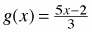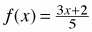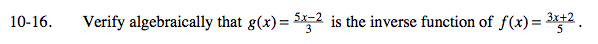### Home > A2C > Chapter 10 > Lesson 10.1.1 > Problem10-16

10-16.

Verify algebraically thatis the inverse function of. Homework Help ✎Substitute g(x) for x in f(x), then simplify. The result should be x.

$\textit{f}(\textit{g}(\textit{x}))=\frac{3\left(\frac{5\textit{x}-2}{3}\right)+2}{5}$